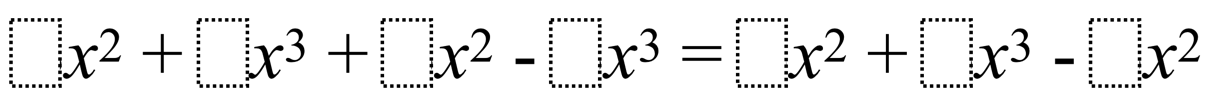Home > High School: Algebra > Arithmetic w/ Polynomials & Rational Expressions > Writing Equivalent Polynomial Expressions

# Writing Equivalent Polynomial Expressions

Directions: Use the digits 1-9, at most one time each, to create a true statement.### Hint

ex. 3+4 = 2+5

3x^2 + 6x^3 + 4x^2 – 1x^2 = 9x^2 + 5x^3 – 2x^2

## Compound Inequalities 2

Directions: Using the digits 1 to 9, at most one time each, make two compound …

1.2.